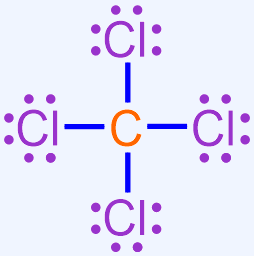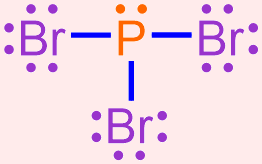# 3.16: Covalent Bonding: Writing Chemical Formulas of Covalent Molecules

$$\newcommand{\vecs}{\overset { \rightharpoonup} {\mathbf{#1}} }$$ $$\newcommand{\vecd}{\overset{-\!-\!\rightharpoonup}{\vphantom{a}\smash {#1}}}$$$$\newcommand{\id}{\mathrm{id}}$$ $$\newcommand{\Span}{\mathrm{span}}$$ $$\newcommand{\kernel}{\mathrm{null}\,}$$ $$\newcommand{\range}{\mathrm{range}\,}$$ $$\newcommand{\RealPart}{\mathrm{Re}}$$ $$\newcommand{\ImaginaryPart}{\mathrm{Im}}$$ $$\newcommand{\Argument}{\mathrm{Arg}}$$ $$\newcommand{\norm}{\| #1 \|}$$ $$\newcommand{\inner}{\langle #1, #2 \rangle}$$ $$\newcommand{\Span}{\mathrm{span}}$$ $$\newcommand{\id}{\mathrm{id}}$$ $$\newcommand{\Span}{\mathrm{span}}$$ $$\newcommand{\kernel}{\mathrm{null}\,}$$ $$\newcommand{\range}{\mathrm{range}\,}$$ $$\newcommand{\RealPart}{\mathrm{Re}}$$ $$\newcommand{\ImaginaryPart}{\mathrm{Im}}$$ $$\newcommand{\Argument}{\mathrm{Arg}}$$ $$\newcommand{\norm}{\| #1 \|}$$ $$\newcommand{\inner}{\langle #1, #2 \rangle}$$ $$\newcommand{\Span}{\mathrm{span}}$$$$\newcommand{\AA}{\unicode[.8,0]{x212B}}$$

Learning Objectives
• Write the chemical formulas of covalent molecules.

In the previous section, the process for drawing a Lewis structure of a covalent molecule was presented and applied.  Recall that covalent bonds are produced when unpaired electrons found within two atoms interact to form a shared pair of electrons.  As a Lewis structure is the result of correctly executing this sharing process, the chemical formula and the chemical name of the corresponding covalent molecule must accurately reflect the information shown in that Lewis structure.  The procedure for using a Lewis structure to determine the chemical formula of a covalent molecule is discussed in the following paragraphs.

## Writing Chemical Formulas of Covalent Molecules

A chemical formula is an abbreviation that indicates both the type and relative proportion of elements that are present in a molecule.  Elemental symbols are used to identify which elements are contained in the molecule, and a whole-number subscript indicates the quantity in which the previous element is present.  If no subscript is written, an unwritten "1" is understood.  The subscripts in the chemical formula of a covalent molecule will vary, based on the identities of the elements that are being combined.

For a covalent molecule, the information represented in its chemical formula must be a direct reflection of its Lewis structure.  Elemental symbols are incorporated into a chemical formula by counting the number of times that each symbol appears in the corresponding Lewis structure.  In order to ensure consistent formatting, the elemental symbol that appears fewer times is written first in a covalent chemical formula, and subscripts are used to indicate how many times each elemental symbol appears in the Lewis structure.  As indicated previously, values of "1" are usually implicitly-understood in chemistry and, therefore, should not be written in a chemical formula.  The subscripts of covalent molecules must not be reduced to the lowest-common ratio of whole numbers, even if it is mathematically-possible to do so, as dividing the subscripts would cause their values to be inconsistent with the number of times that each elemental symbol appears in the Lewis structure.

For example, consider the Lewis structure shown below, which represents a covalent molecule that is formed when fluorine and sulfur bond with one another.This Lewis structure contains two fluorine atoms and one sulfur atom.  As there are fewer sulfur atoms in the Lewis structure, the elemental symbol "S" is written first in the corresponding chemical formula, and fluorine's elemental symbol, "F," is written second.  No subscript should be written on sulfur's elemental symbol, as values of "1" should not be explicitly-written in a chemical formula.  A subscript of "2" should be written on fluorine's elemental symbol.  The resultant chemical formula, SF2, accurately summarizes the information in the Lewis structure shown above and, therefore, is the chemically-correct formula for this covalent molecule.

Example $$\PageIndex{1}$$

Use the Lewis structure shown below to write the chemical formula for the covalent molecule that is formed when carbon and chlorine bond with one another.Solution

This Lewis structure contains one carbon atom and four chlorine atoms.  As there are fewer carbon atoms in the Lewis structure, the elemental symbol "C" is written first in the corresponding chemical formula, and chlorine's elemental symbol, "Cl," is written second.  No subscript should be written on carbon's elemental symbol, as values of "1" should not be explicitly-written in a chemical formula.  A subscript of "4" should be written on chlorine's elemental symbol.  The resultant chemical formula, CCl4, accurately summarizes the information in the Lewis structure shown above and, therefore, is the chemically-correct formula for this covalent molecule.

Exercise $$\PageIndex{1}$$

Use the Lewis structure shown below to write the chemical formula for the following covalent molecule that is formed when bromine and phosphorus bond with one another.